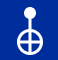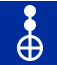# Introduction to Quantum Operations

Quantum operations are operations use to manipulate quantum bits (or qubits, for short) in a quantum circuit. They include quantum gates (like the Pauli-Z gate), as well as operations that are not quantum gates (like the IF operation). This is a short introduction to some of the gates I’ll be using in the future in Quantum Algorithms.

# Phase Gates

## The T Gate

The T gate applies a 𝛑/4 phase to a qubit. It is also sometimes referred to as a 𝛑/8 gate. This gate is not a Clifford gate. The IBM Quantum Experience symbol for this gate is:

## The S gate

Next, we have the S gate. This gate applies a phase of i to the ⎪1⟩ state. In case you forgot, i is the imaginary unit and is defined as i = √-1. In general, it applies a phase of 𝛑/2. This gate is also sometimes called the phase gate, or P gate. The S gate is a Clifford gate. The IBM Quantum Experience symbol for it is:

## The Pauli Z gate

The Pauli Z gate is the identity of the ⎪0⟩ state (it does nothing/has no effect), and flips the sign of the ⎪1⟩ state. So if it was negative, after applying the Pauli Z gate, the sign would now be positive, and vice versa. It basically flips the ⎪−⟩ and ⎪+⟩ states. The symbol for it is:

## The Sdg gate

This gate is the inverse of the S gate; it “reverses” the S gate by inducing a phase of −𝛑/2. The “dg” part represents the symbol †, or dagger. It is also a Clifford gate. The symbol for it is:

## The Tdg gate

Similarly, this gate is the inverse of the T gate; it “reverses” the T gate by inducing a phase of −𝛑/4. This gate is not a Clifford gate. It’s symbol is:

## The U1 gate

The U1 gate induces a phase of e^iθ to the ⎜1⟩ state. For some values of the angle θ theta (in radians), this gate is equivalent to other gates. For example, when θ = 𝛑 radians, this is equivalent to the Pauli Z gate, and when θ = 𝛑/4, this is equivalent to the T gate. This gate is a diagonal gate. The symbol for it is:

# Classical Gates

## The Pauli X gate (also called NOT gate)

The Pauli X gate is a relatively simple gate. It is used interchangeably with the NOT gate. It essentially flips the state ⎪0⟩ to ⎪1⟩ and vice versa. The symbol representation for it is:

## The CX gate

This gate is also known as the controlled-X gate, or the controlled-NOT gate, and it acts on a pair or qubits: one of them as the “control” and the other as the “target.” It performs a Pauli X / NOT operation on the “target” if the “control” is in the state ⎪1⟩. If the “control” qubit is in a superposition, it entangles the “target” and “control” qubits. The symbol for it is:CX / Controlled-X / Controlled-NOT gate

## The CCX gate

The CCX is — you guessed it—the double Controlled-X (or double Controlled-NOT) gate and is sometimes also called the Toffoli gate. It’s a bit like the CX gate but the main difference is that there are two “control” qubits instead of one. It behaves similarly, but only performs a Pauli X operation on the “target” qubit when both the “control” qubits are in the state⎪1⟩. The symbol for it is:CCX / double Controlled-X / double NOT gate

## The SWAP gate

The SWAP gate is a very simple gate. It does exactly what its name suggests it does: it swaps the states of two qubits. Note that when both qubits are in the same state, then it has no effects. It’s symbol is:

## The CSWAP gate

This gate is the Controlled-SWAP gate. It’s similar to the CCX gate, but it has two “target” qubits and one “control” qubit, as opposed to two “control” qubits and one “target” qubit for the CCX. It is also called the Fredkin gate. This gate swaps the states of the “target” qubits if the “control” qubit is in the state⎪1⟩. The symbol for it is:

# Non-unitary Operations

## The Barrier operation

The Barrier operation forms a barrier between successive operations, which prevents compilers from combining gates, since combining gates makes your quantum program more efficient. It is also useful for visualizing your circuit. This what its symbol looks like

## The Reset operation

The Reset operation resets a qubit to the state⎪0⟩, regardless of what state it was before. This operation is not reversible, which means you cannot tell what the input(s) were based on the output(s). The symbol for it is:

## The IF operation

The IF operation is a conditional operation, in other words, it does X only when Y. For example, IF Steven is the Imposter, then Steven if SUS. Or, in the context of a quantum circuit, if qubit 1 is in the state⎪1⟩, then apply a Pauli X gate to qubit 2. Its symbol looks like this:

## The Measurement operation

The Measurement operation measures the state of a qubit, therefore collapsing any superpositions. It can also be combined with gates to implement any measurement. Like the Reset operation, this operation is also not reversible. This is the symbol:

# Other Quantum gates

## The Hadamard gate

The Hadamard gate is named after Jacques Hadamard, and is also sometimes called the H gate for short. This gate rotates the states⎪0⟩ and⎪1⟩ to⎪+⟩ and⎪−⟩, respectively, which is useful for creating superpositions of qubits. Its symbol looks like this:

## The RX gate

The RX gate performs e^iθX, which corresponds to a rotation of the qubit state around the X-axis by the given angle theta θ on the Bloch Sphere. A simple way to remember what this gate does is to think of the R as “rotation,” and the X as “x-axis.” This is what the RX gate symbol is:

## The RY gate

Likewise, the RY gate performs e^iθY, which corresponds to a rotation of the qubit state around the Y-axis by the given angle theta θ on the Bloch Sphere. This gate does not create complex amplitutdes. A simple way to remember what this gate does is to think of the R as “rotation,” and the Y as “y-axis.” This is what the RY gate symbol looks like

## The RZ gate

As above, the RZ gate is like the RX and RY gates. It corresponds to a rotation of the qubit state around the Z-axis by the given angle theta θ on the Bloch Sphere. This gate is a diagonal gate. A simple way to remember what this gate does is to think of the R as “rotation,” and the Zas “z-axis.” This is the RZ gate symbol:

## The U3 gate

The U3 gate allows you to construct any single-qubit gate, by taking in three parameters: theta θ, phi 𝞍, and lambda ƛ. Think of it as the custom gate creator. Any gate created has a duration of one unit of gate time, in other words, it’s a one-time only thing. This is what it’s symbol looks like:

## The Pauli Y gate

The Pauli Y gate is equivalent to a rotation of 𝛑 radians around the Y-axis, as well as applying the Pauli X and Pauli Y gates up to a certain angle. The symbol for it is:

## The U2 gate

The U2 gate takes in two parameters, theta θ and phi 𝞍, and controls two different rotations within itself. Like the U3 gate above, it can be seen as a “custom gate creator.” It also has a duration of one unit of gate time, like the U3 gate. The symbol for it looks like this:

## The CH gate

The CH gate is the Controlled-Hadamard gate. As with all controlled gates, it has a “target” qubit and a “control” qubit. This gate performs the Hadamard gate operation on the “target” qubit only when the “control” qubit is in the ⎜1⟩ state. This is its symbol:

## The CY gate

The CY gate is the Controlled-Y gate. It takes two input qubits, one “control” and one “target,” and performs the Y gate operation on the “target” qubit only when the “control” qubit is in the⎜1⟩ state. The symbol for it is:

## The CZ gate

The CZ gate is the Controlled-Z gate. Like the CH and CY gates above, it also takes two inputs, a “control” and “target” qubit. This gate performs the Z gate operation on the “target” qubit whenever the “control” qubit is in the state⎜1⟩. This gate has a nice symmetry to it; swapping the “control” and “target” qubits does not change the output. Its symbol is:

## The CRX gate

The CRX gate is the Controlled-RX gate. Like all controlled gates it takes a “target” and “control” qubit as its inputs. This gate performs the RX gate operation only when the “control” is in⎜1⟩ state. Its symbol is:

## The CRY gate

The misleading name of this gate is a travesty. It does not, as the name suggests, make you cry. Rather, it is the Controlled-RY gate, which takes in two inputs (“control” and “target” qubits), and performs a the RY gate operation when the “control” qubit is in the⎜1⟩ state. The symbol for it is:

## The CRZ gate

The CRZ is the Controlled-Z gate. Like the CRX and CRY gates above, it takes in a “control” and “target” qubit as inputs. It performs the RZ gate operation when the “control” is in the state⎜1⟩. The symbol for it is:

## The CU1 gate

This gate is the Controlled-1 gate. This gate also has two inputs (you know what they are now), and it performs the U1 gate operation on the “target” qubit when the “control” qubit is in the⎜1⟩ state. Its symbol is:

## The CU3 gate

The CU3 gate is the Controlled-U3 gate. This gate takes in a “control” and “target” qubit, and performs the U3 gate operation on the “target” qubit when the “control” qubit is in the state⎜1⟩. Its symbol is:

## The RXX gate

The RXX gates performs the operation ^(-iθ/2X⊕ X) on the input qubit. The symbol for this gate is:

## The RZZ gate

Finally, our last gate is the RZZ gate. This gate takes in one input, an angle theta θ expressed in radians, and it acts on two qubits. In addition, it is symmetric, meaning that swapping the qubits it acts on has no effect on the output. Its symbol is:

# Conclusion

So hopefully you found this Introduction to Quantum Operations article very helpful. I’ll be using some of these gates later when creating some basic (and more advanced) Quantum Algorithms, so this article should prepare you for when you see these gates in an actual circuit. Click here to read more about Quantum Operations.

--

--## Max Cui

A person who is passionate about technologies such as Quantum Computing, Blockchain, and Machine Learning/AI. Currently working on a QCNN for Image Recognition!